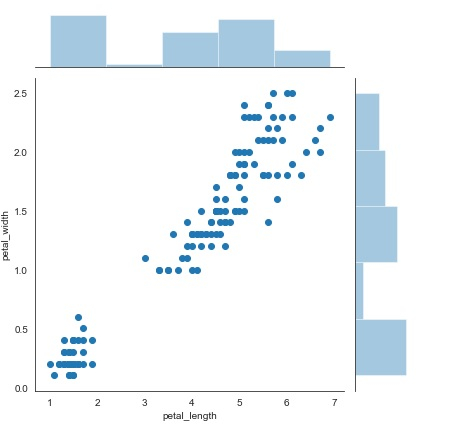# How can Seaborn library be used to display a Scatter Plot in Python?

Visualizing data is an important step since it helps understand what is going on in the data without actually looking at the numbers and performing complicated computations. Seaborn is a library that helps in visualizing data.

Scatter plot shows the distribution of data as data points that are spread/scattered on the graph. It uses dots to represents values of a dataset, which are numeric in nature. The position of every dot on the horizontal and vertical axis denotes the value for a single data point.

They help understand the relationship between two variables. Let us understand how this can be achieved using Seaborn library in Python −

## Example

import seaborn as sb
from matplotlib import pyplot as plt
sb.jointplot(x = 'petal_length',y = 'petal_width',data = df)
plt.show()

## Output## Explanation

• The required packages are imported.
• The input data is ‘iris_data’ which is loaded from the scikit learn library.
• This data is stored in a dataframe.